Скачать презентацию 55 Grade Hoops Add Subtract Multiply Divide

• Количество слайдов: 55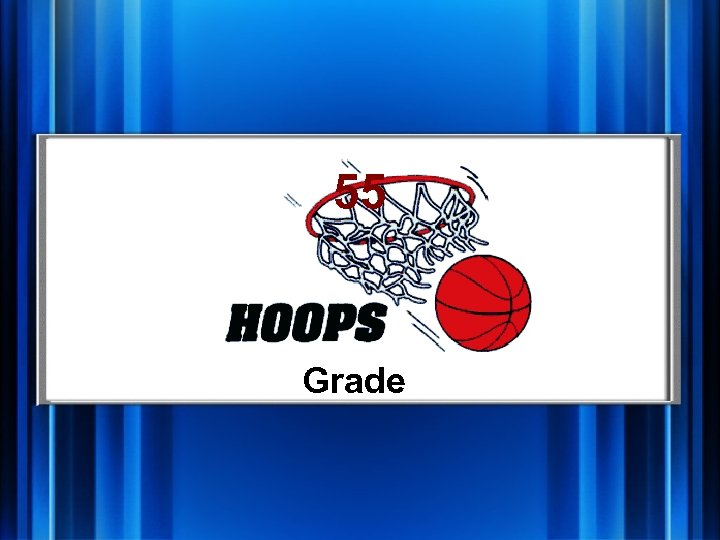55 Grade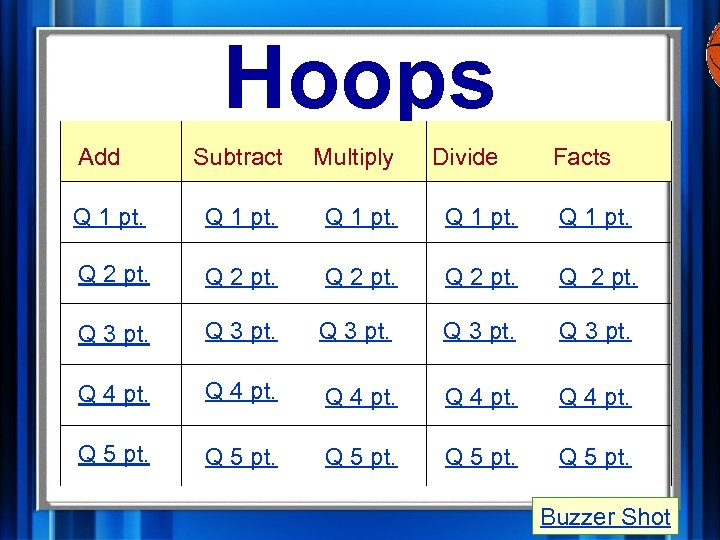Hoops Add Subtract Multiply Divide Facts Q 1 pt. Q 2 pt. Q 3 pt. Q 4 pt. Q 5 pt. Buzzer Shot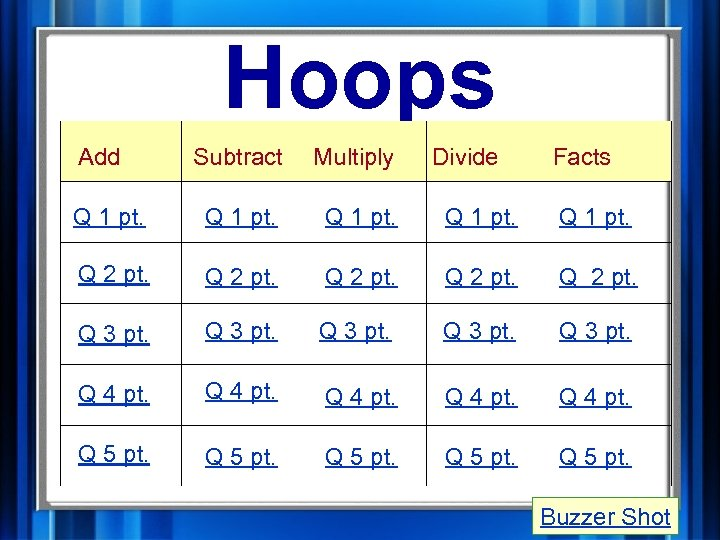Hoops Add Subtract Multiply Divide Facts Q 1 pt. Q 2 pt. Q 3 pt. Q 4 pt. Q 5 pt. Buzzer Shot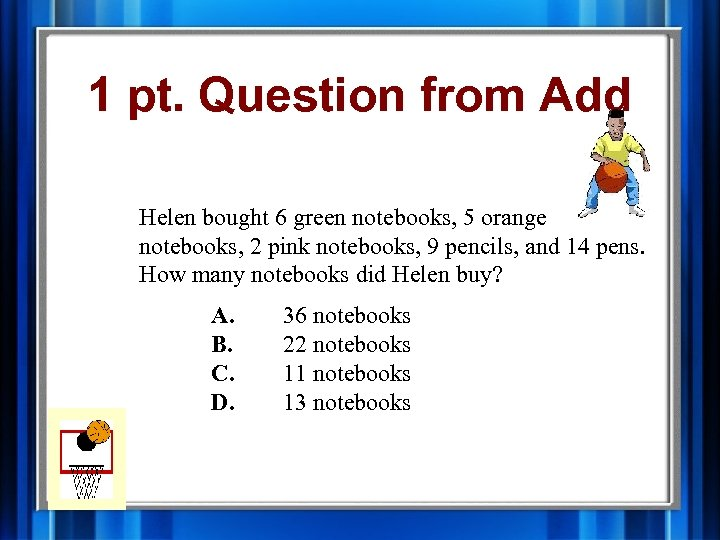1 pt. Question from Add Helen bought 6 green notebooks, 5 orange notebooks, 2 pink notebooks, 9 pencils, and 14 pens. How many notebooks did Helen buy? A. B. C. D. 36 notebooks 22 notebooks 11 notebooks 13 notebooks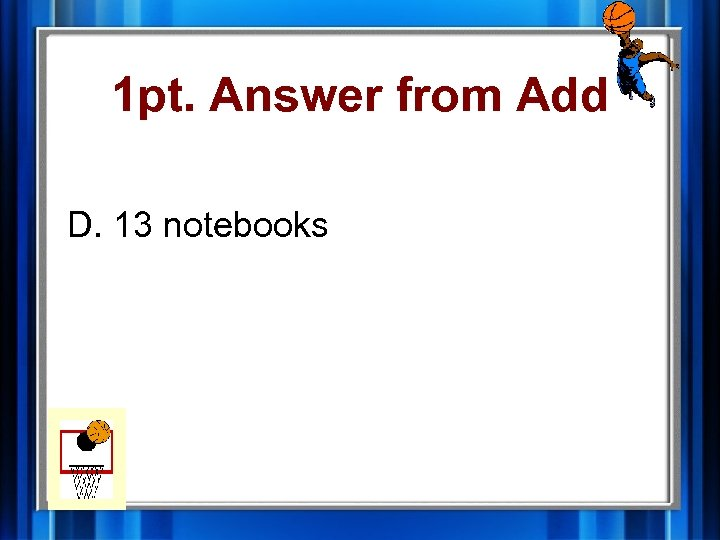1 pt. Answer from Add D. 13 notebooks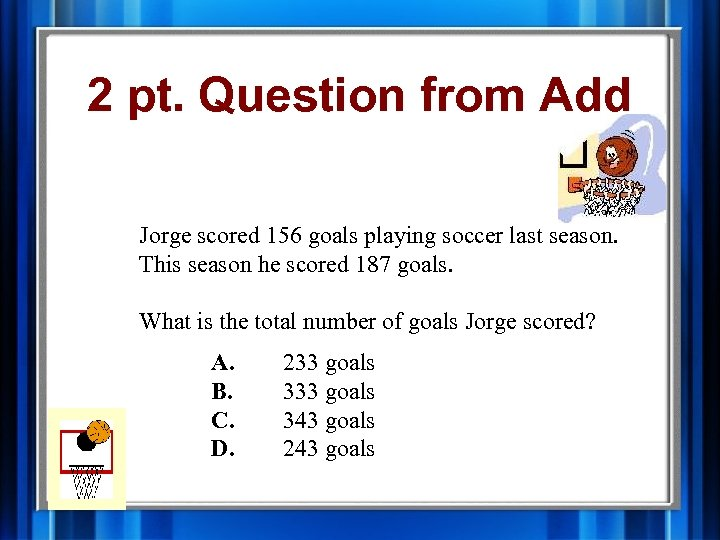2 pt. Question from Add Jorge scored 156 goals playing soccer last season. This season he scored 187 goals. What is the total number of goals Jorge scored? A. B. C. D. 233 goals 343 goals 243 goals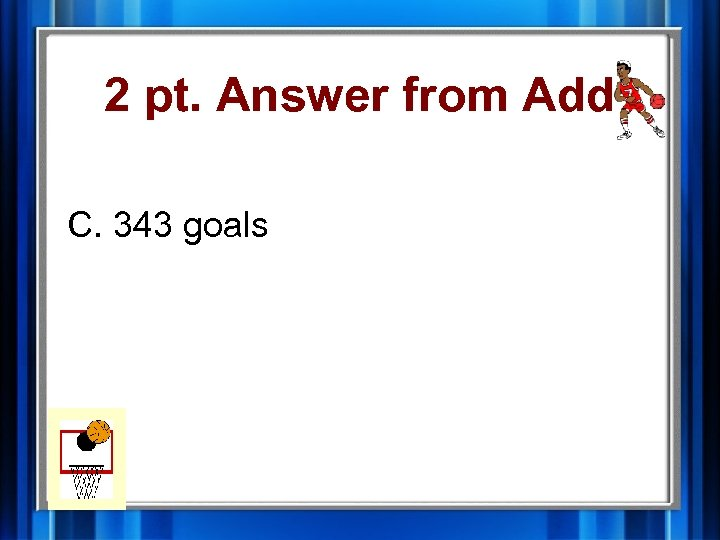2 pt. Answer from Add C. 343 goals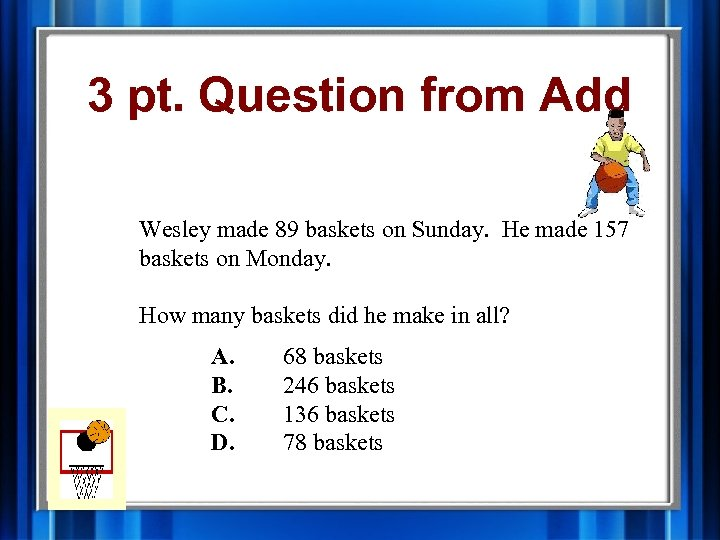3 pt. Question from Add Wesley made 89 baskets on Sunday. He made 157 baskets on Monday. How many baskets did he make in all? A. B. C. D. 68 baskets 246 baskets 136 baskets 78 baskets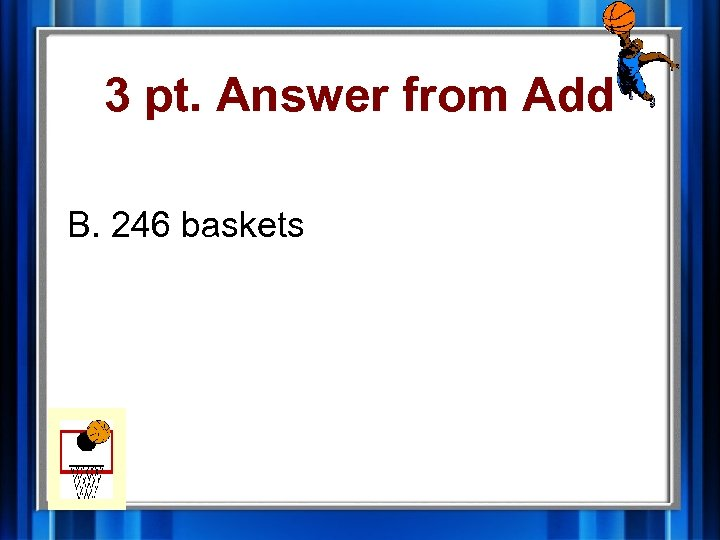3 pt. Answer from Add B. 246 baskets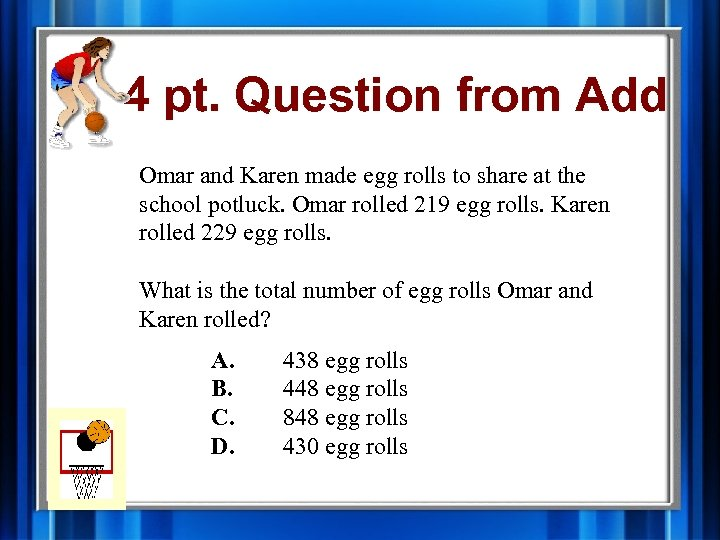4 pt. Question from Add Omar and Karen made egg rolls to share at the school potluck. Omar rolled 219 egg rolls. Karen rolled 229 egg rolls. What is the total number of egg rolls Omar and Karen rolled? A. B. C. D. 438 egg rolls 448 egg rolls 848 egg rolls 430 egg rolls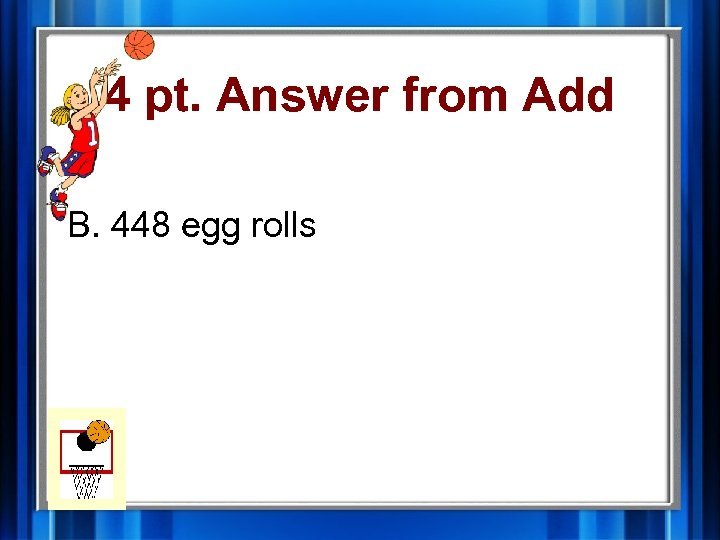4 pt. Answer from Add B. 448 egg rolls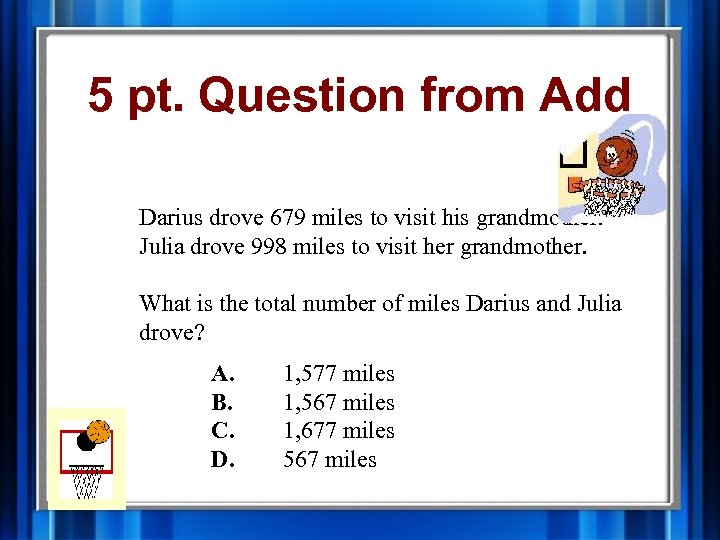5 pt. Question from Add Darius drove 679 miles to visit his grandmother. Julia drove 998 miles to visit her grandmother. What is the total number of miles Darius and Julia drove? A. B. C. D. 1, 577 miles 1, 567 miles 1, 677 miles 567 miles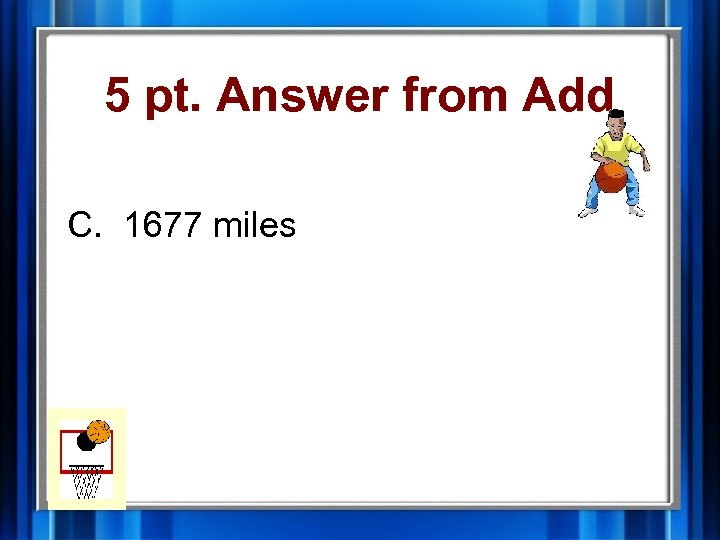5 pt. Answer from Add C. 1677 miles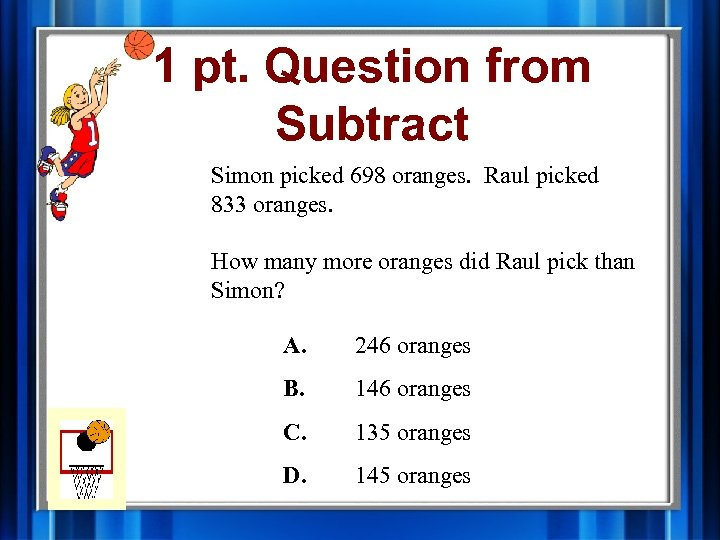1 pt. Question from Subtract Simon picked 698 oranges. Raul picked 833 oranges. How many more oranges did Raul pick than Simon? A. 246 oranges B. 146 oranges C. 135 oranges D. 145 oranges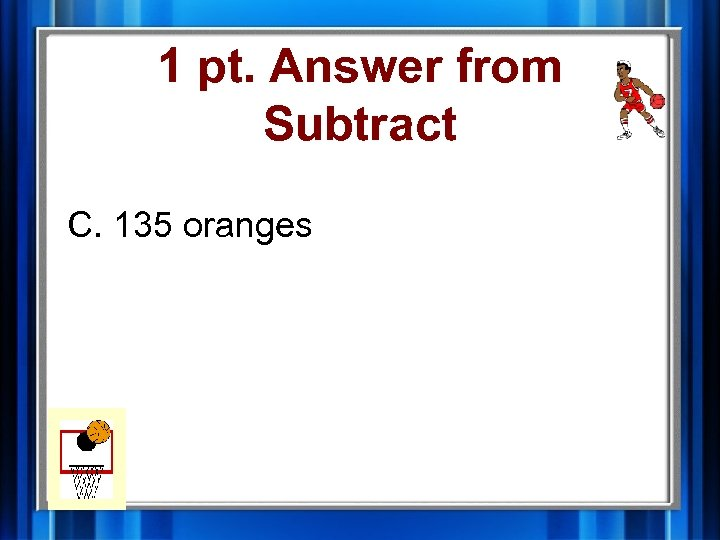1 pt. Answer from Subtract C. 135 oranges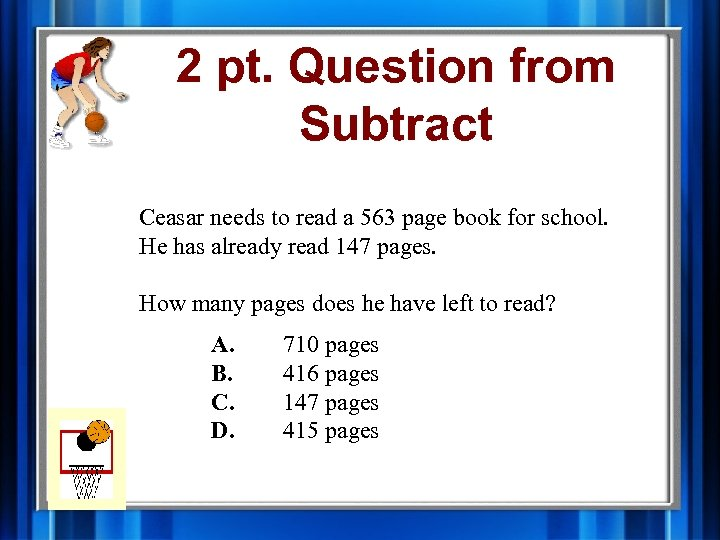2 pt. Question from Subtract Ceasar needs to read a 563 page book for school. He has already read 147 pages. How many pages does he have left to read? A. B. C. D. 710 pages 416 pages 147 pages 415 pages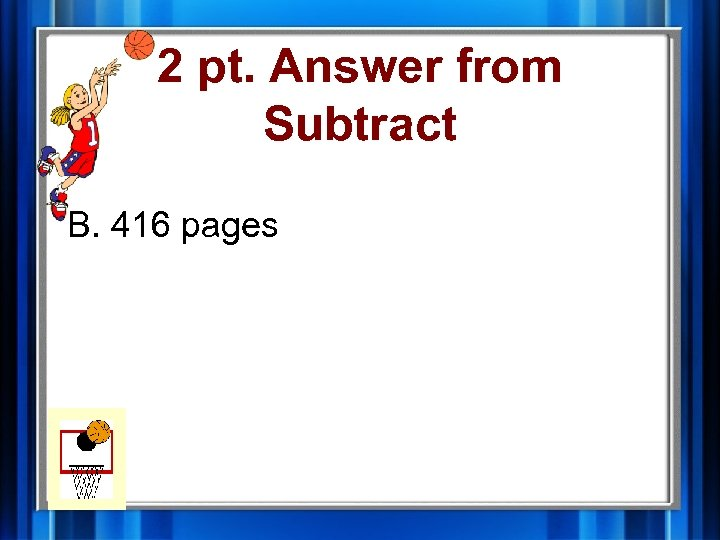2 pt. Answer from Subtract B. 416 pages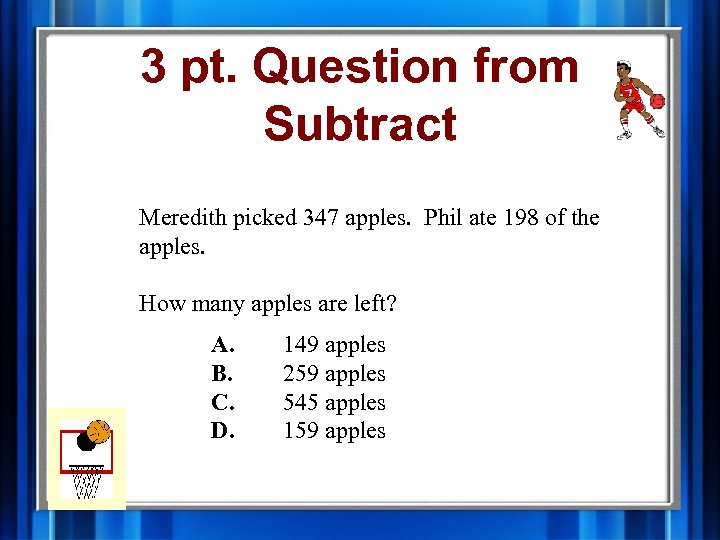3 pt. Question from Subtract Meredith picked 347 apples. Phil ate 198 of the apples. How many apples are left? A. B. C. D. 149 apples 259 apples 545 apples 159 apples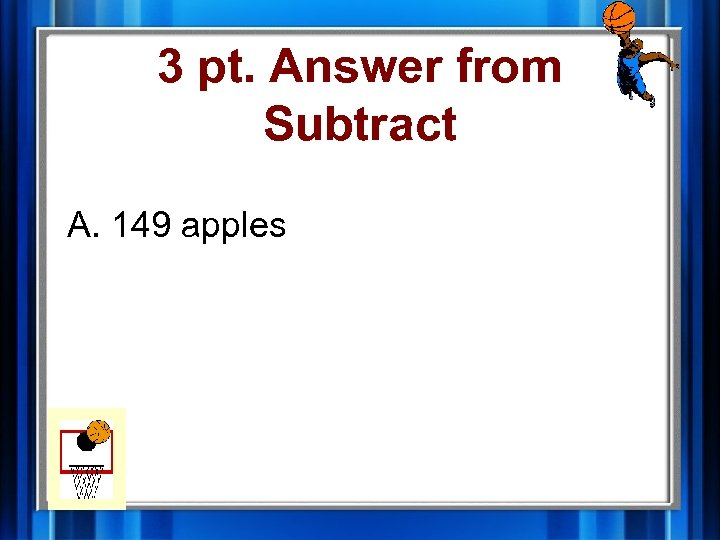3 pt. Answer from Subtract A. 149 apples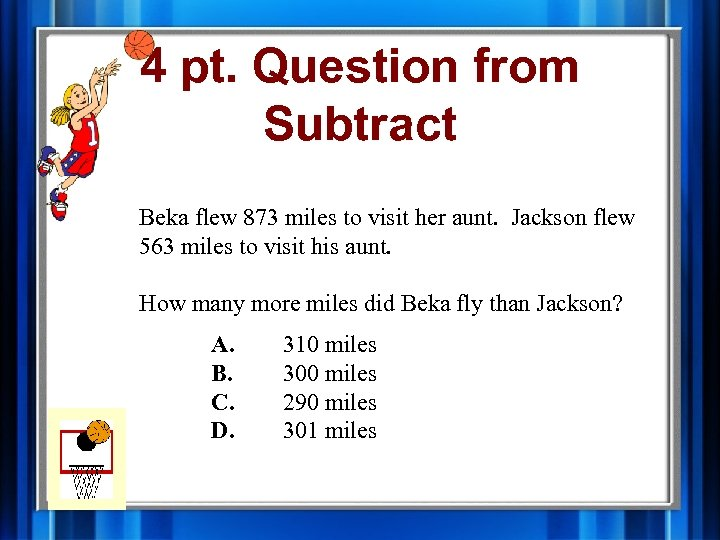4 pt. Question from Subtract Beka flew 873 miles to visit her aunt. Jackson flew 563 miles to visit his aunt. How many more miles did Beka fly than Jackson? A. B. C. D. 310 miles 300 miles 290 miles 301 miles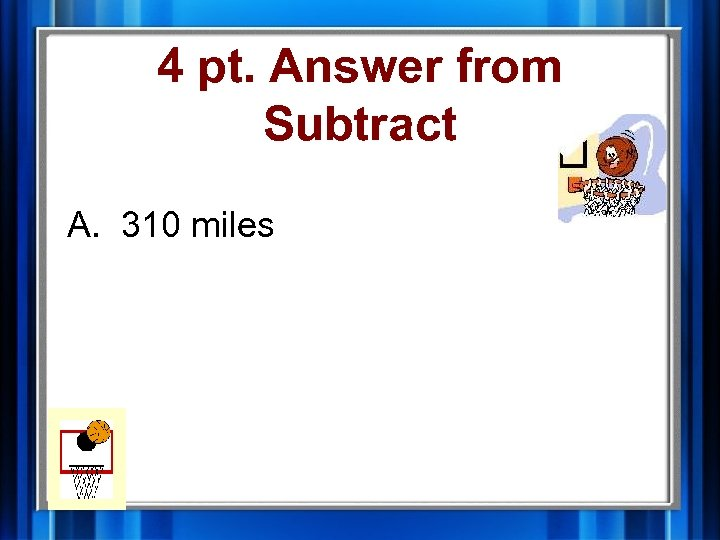4 pt. Answer from Subtract A. 310 miles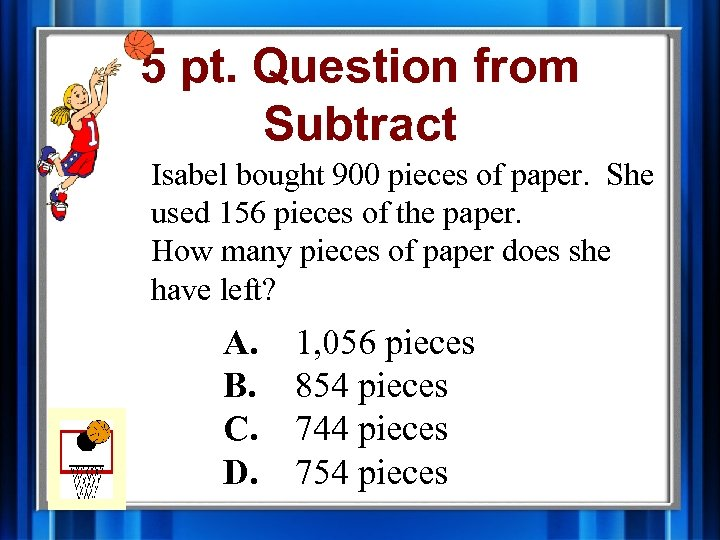5 pt. Question from Subtract Isabel bought 900 pieces of paper. She used 156 pieces of the paper. How many pieces of paper does she have left? A. B. C. D. 1, 056 pieces 854 pieces 744 pieces 754 pieces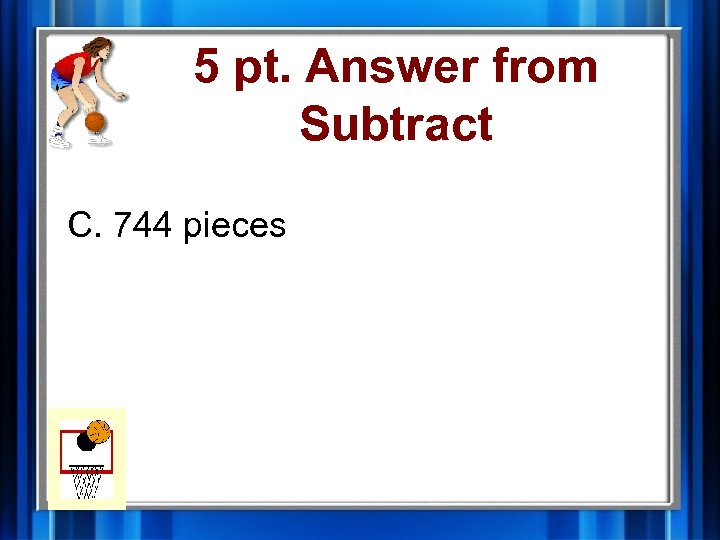5 pt. Answer from Subtract C. 744 pieces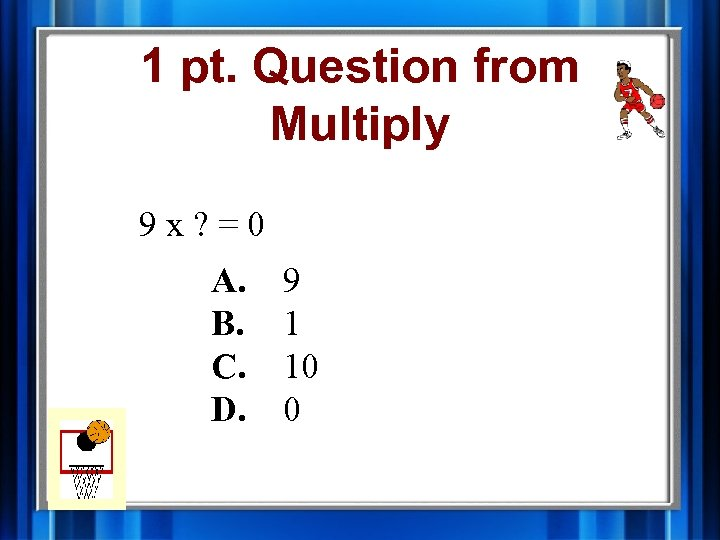1 pt. Question from Multiply 9 x? =0 A. B. C. D. 9 1 10 0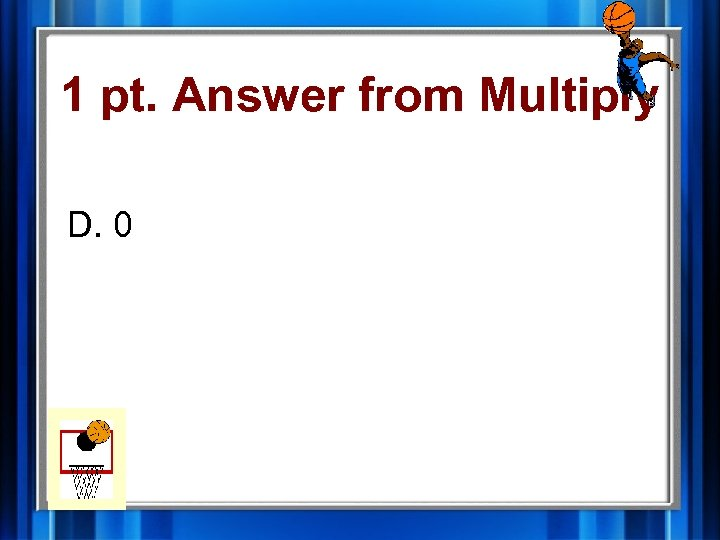1 pt. Answer from Multiply D. 0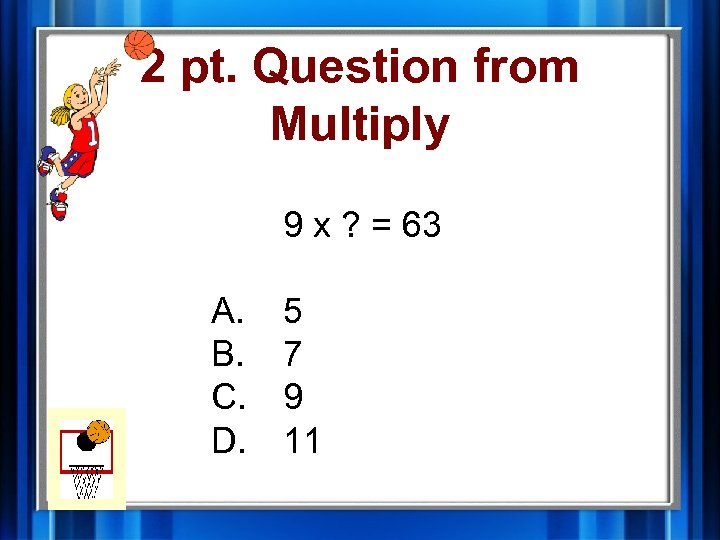2 pt. Question from Multiply 9 x ? = 63 A. B. C. D. 5 7 9 11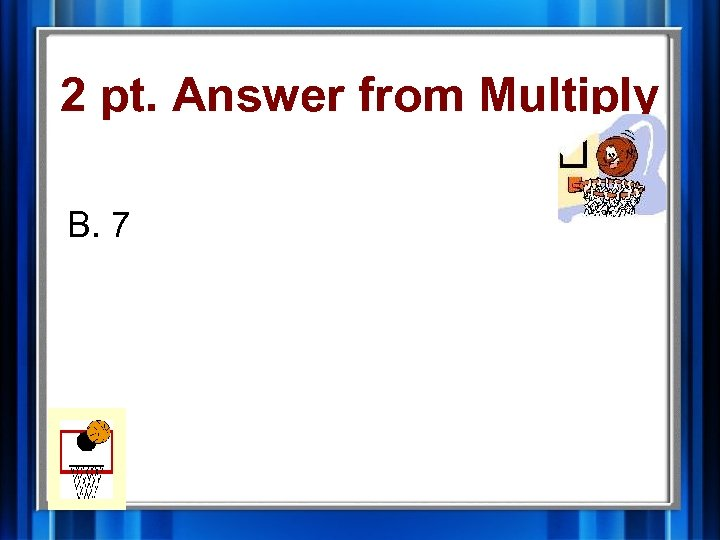2 pt. Answer from Multiply B. 7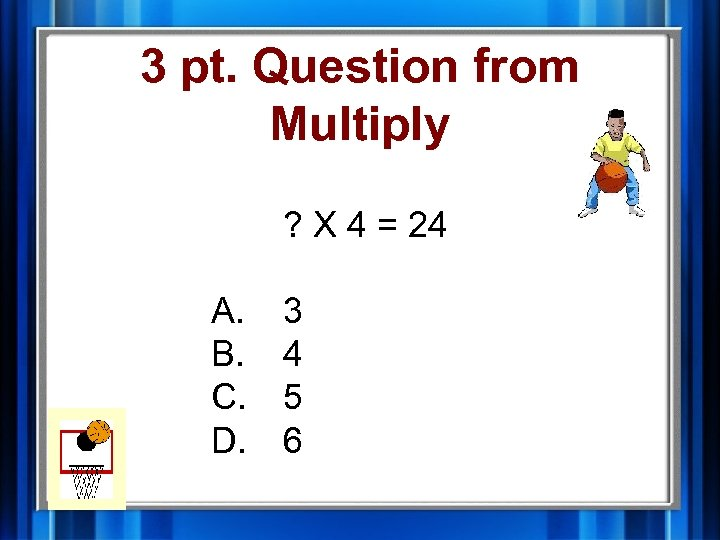3 pt. Question from Multiply ? X 4 = 24 A. B. C. D. 3 4 5 6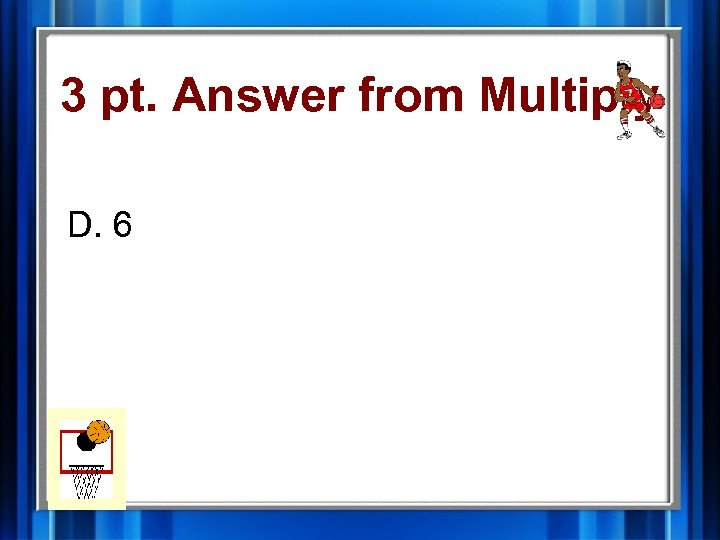3 pt. Answer from Multiply D. 6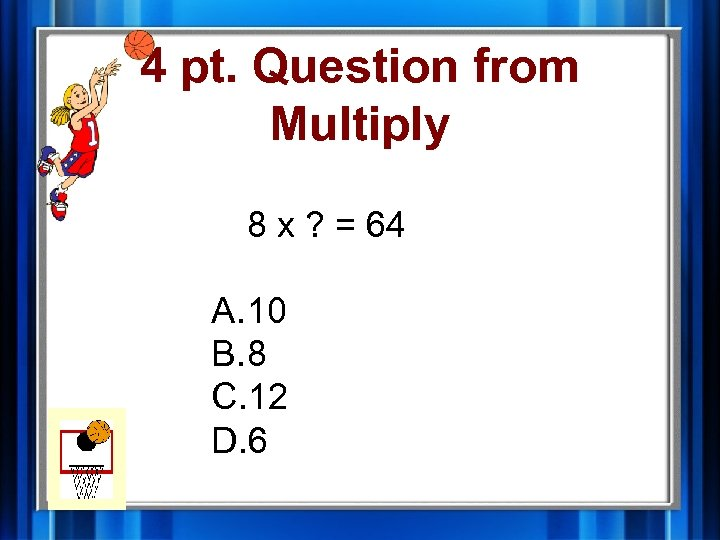4 pt. Question from Multiply 8 x ? = 64 A. 10 B. 8 C. 12 D. 6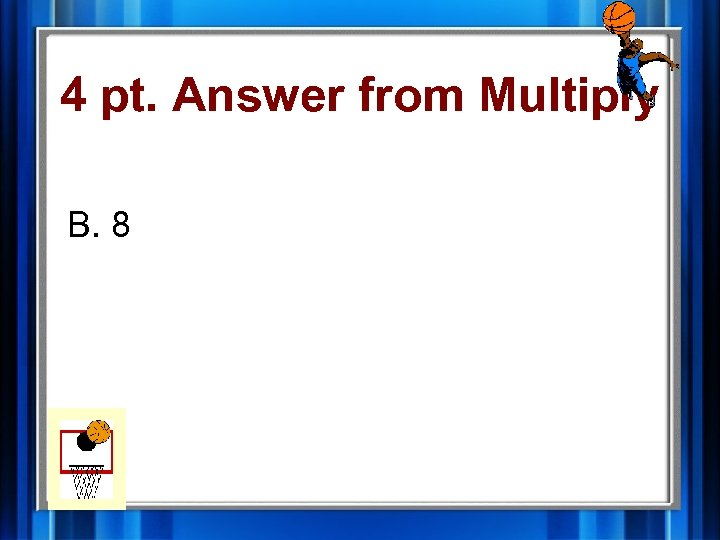4 pt. Answer from Multiply B. 8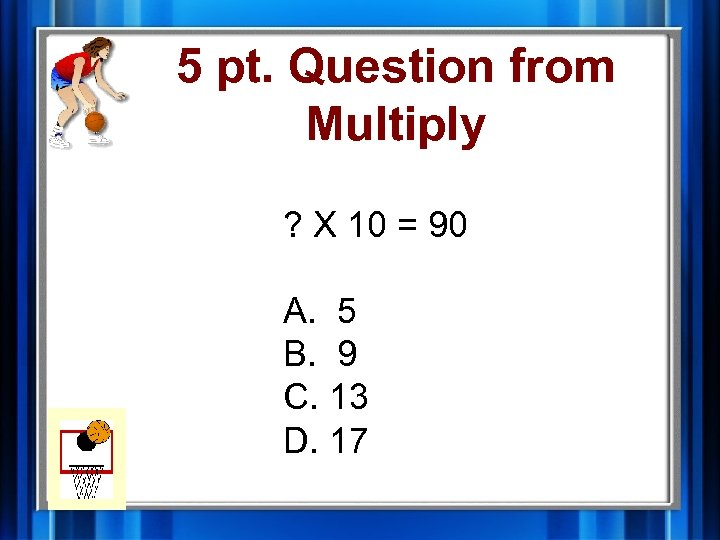5 pt. Question from Multiply ? X 10 = 90 A. 5 B. 9 C. 13 D. 17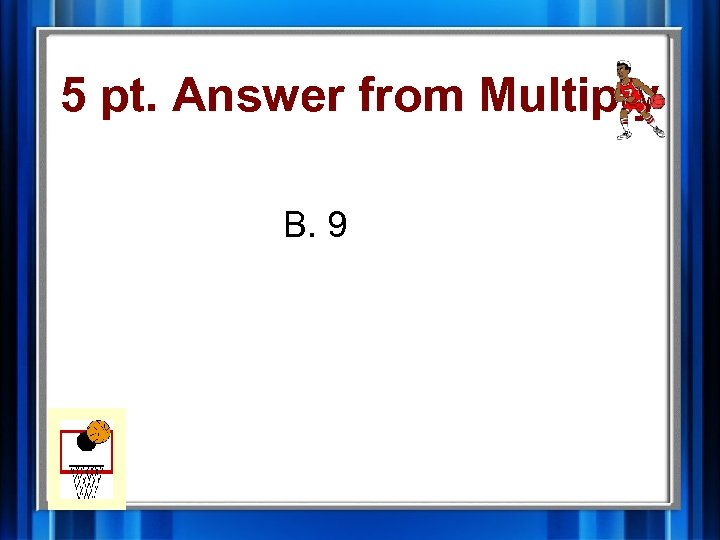5 pt. Answer from Multiply B. 9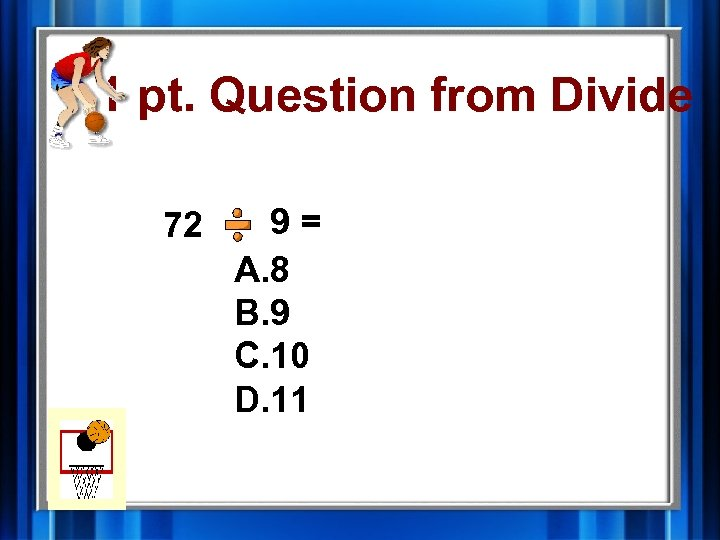1 pt. Question from Divide 72 9= A. 8 B. 9 C. 10 D. 11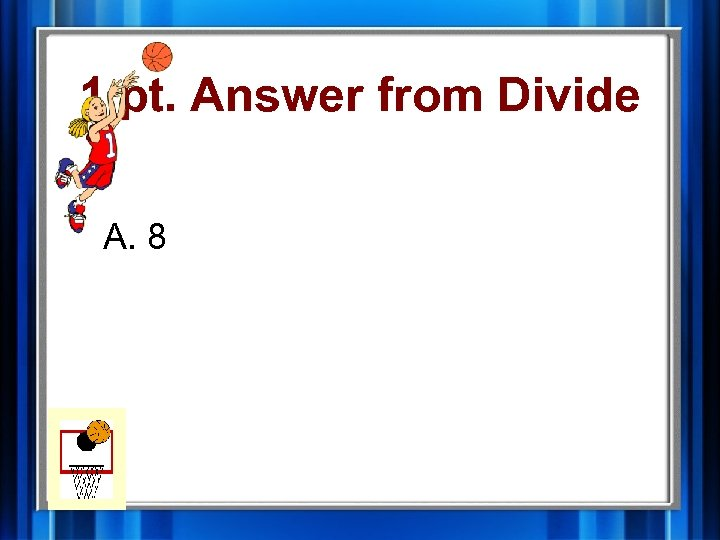1 pt. Answer from Divide A. 8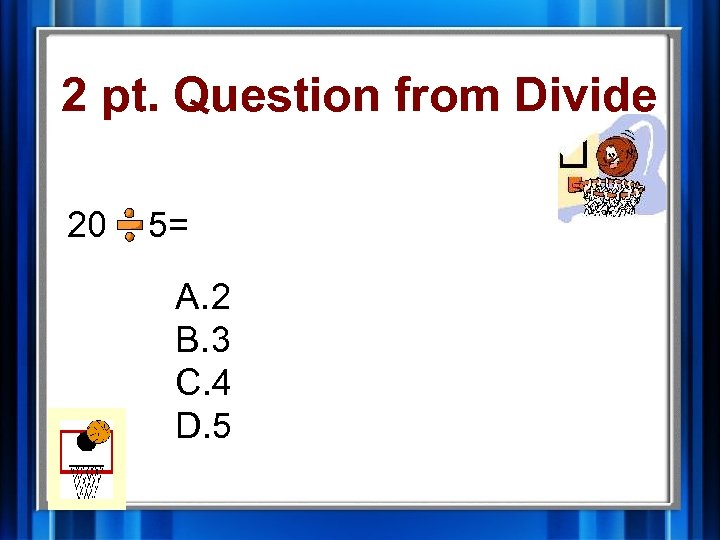2 pt. Question from Divide 20 5= A. 2 B. 3 C. 4 D. 5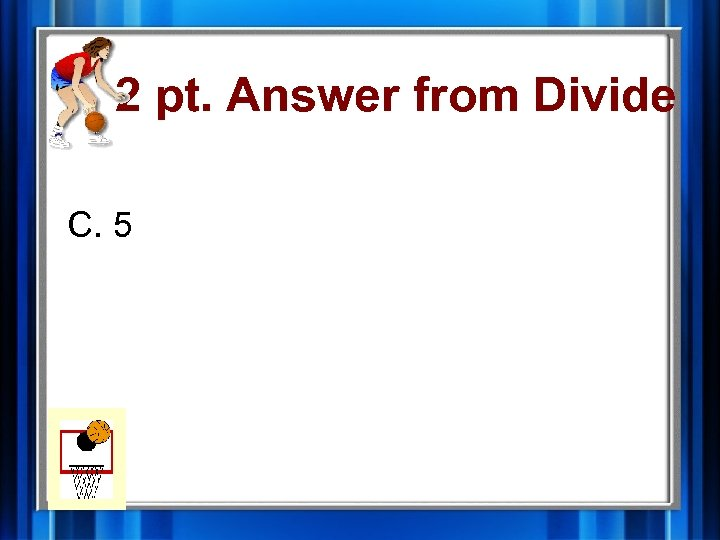2 pt. Answer from Divide C. 5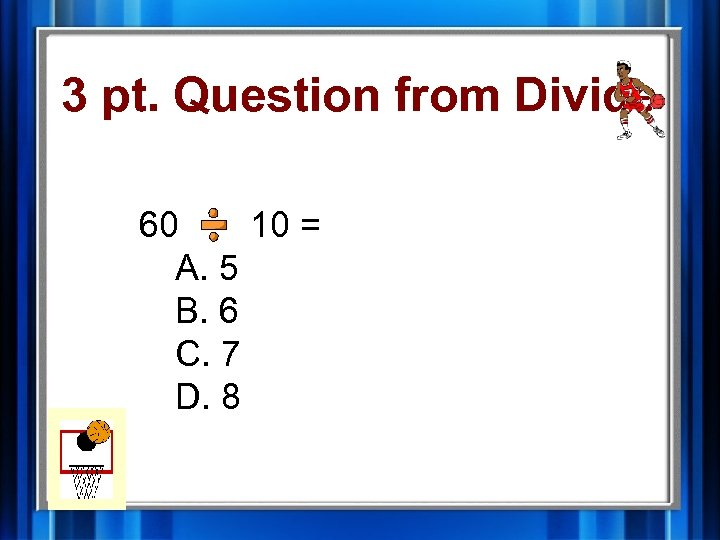3 pt. Question from Divide 60 10 = A. 5 B. 6 C. 7 D. 8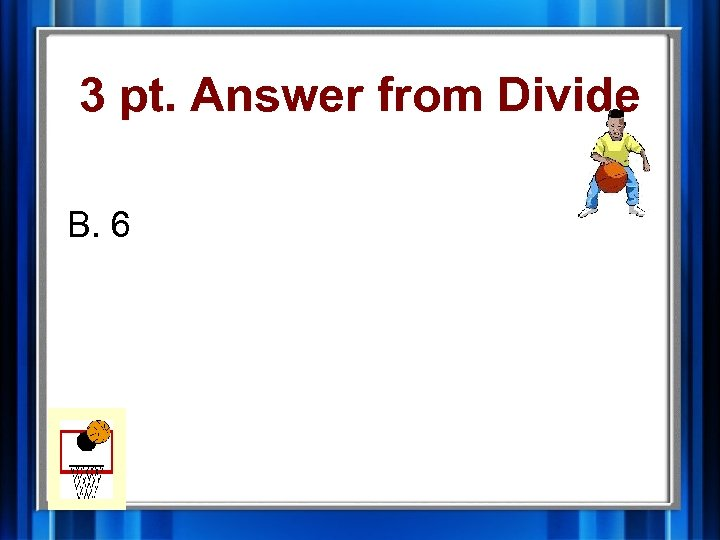3 pt. Answer from Divide B. 6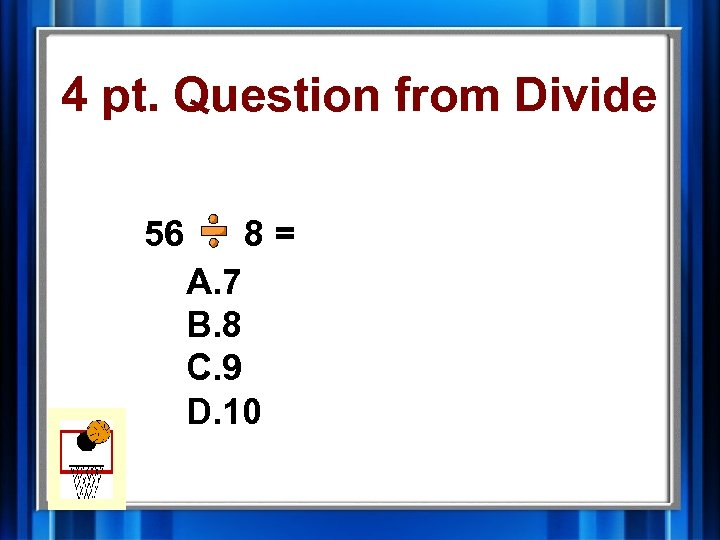4 pt. Question from Divide 56 8= A. 7 B. 8 C. 9 D. 10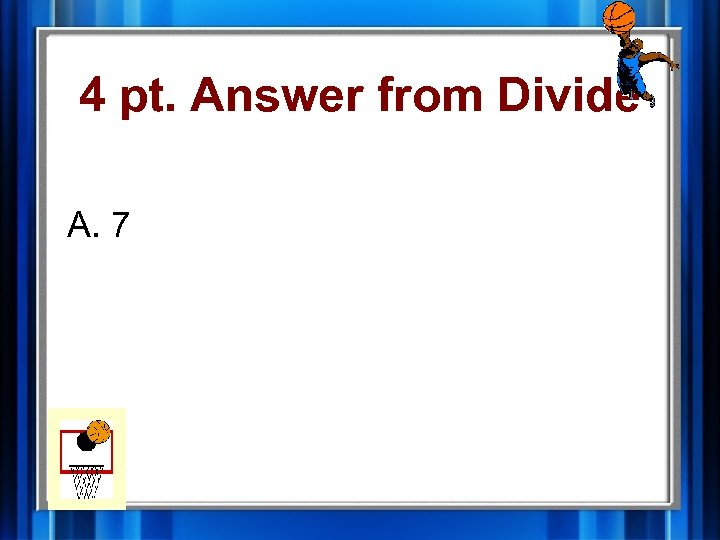4 pt. Answer from Divide A. 7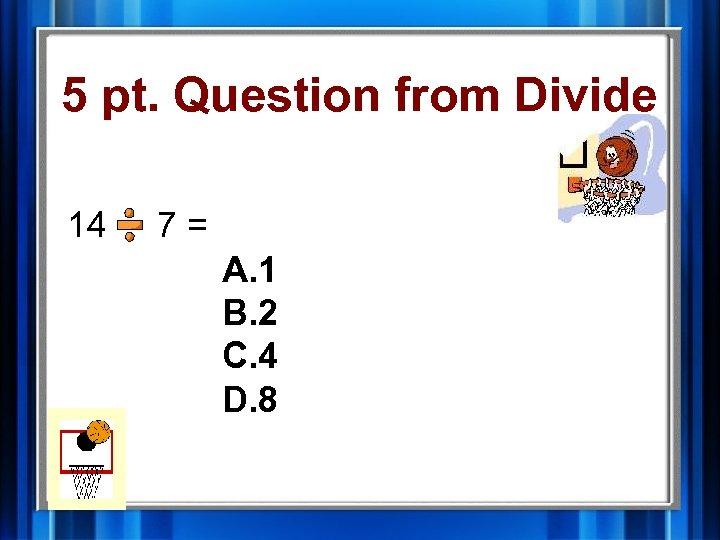5 pt. Question from Divide 14 7= A. 1 B. 2 C. 4 D. 8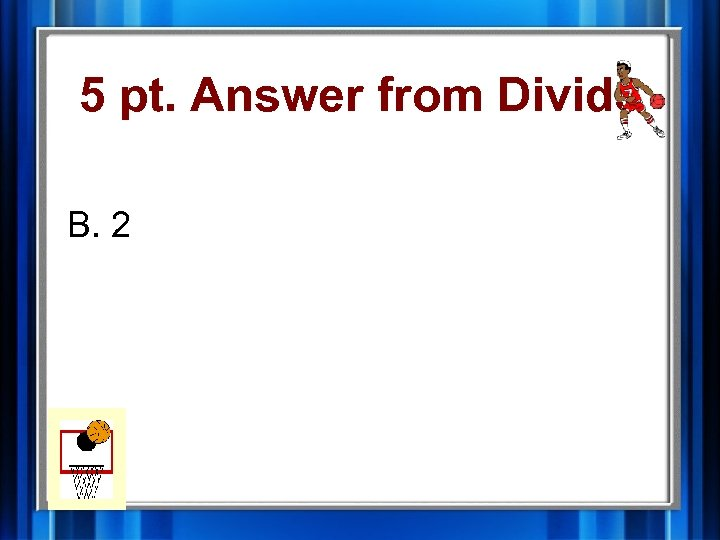5 pt. Answer from Divide B. 2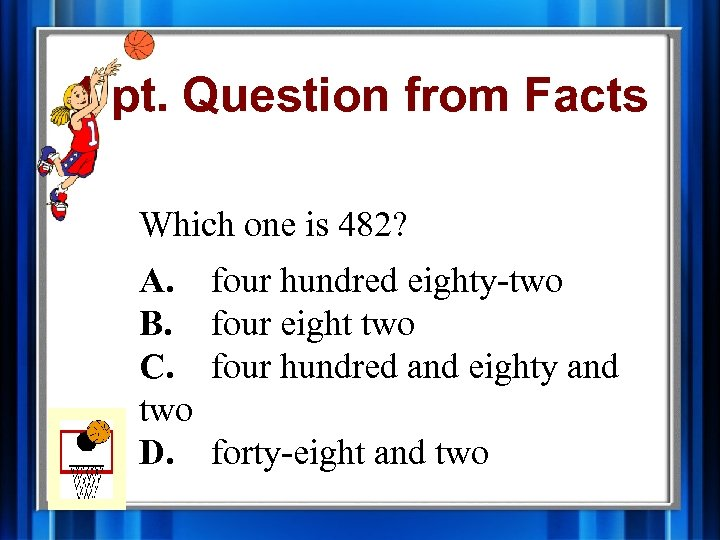1 pt. Question from Facts Which one is 482? A. B. C. two D. four hundred eighty-two four eight two four hundred and eighty and forty-eight and two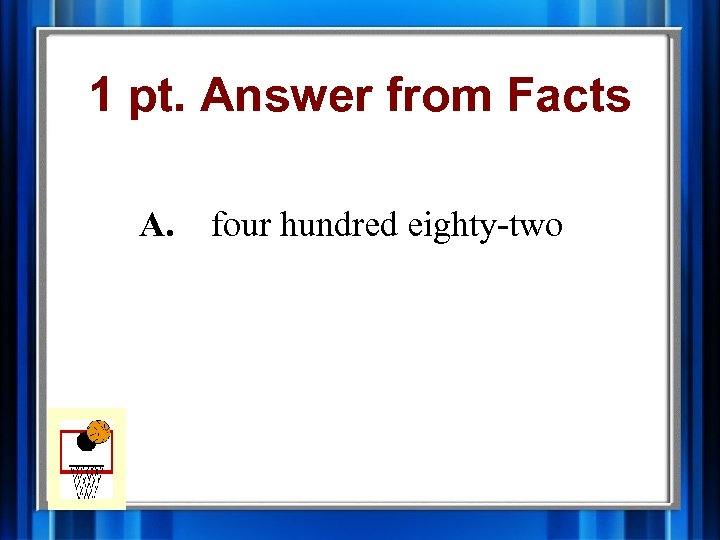1 pt. Answer from Facts A. four hundred eighty-two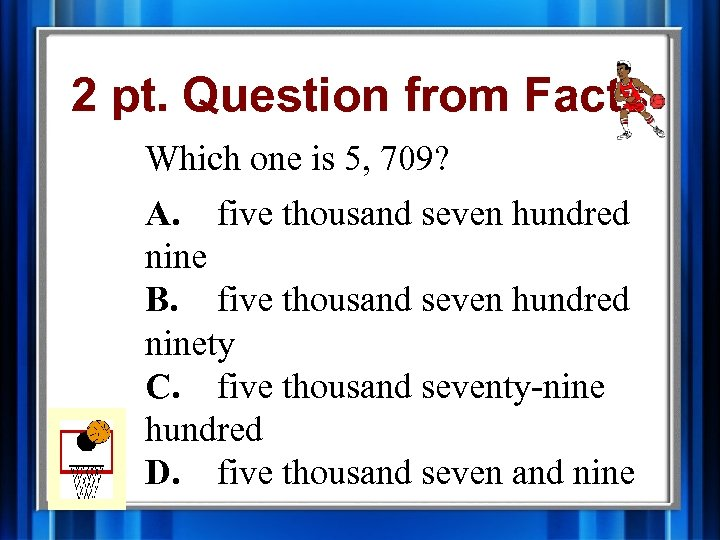2 pt. Question from Facts Which one is 5, 709? A. five thousand seven hundred nine B. five thousand seven hundred ninety C. five thousand seventy-nine hundred D. five thousand seven and nine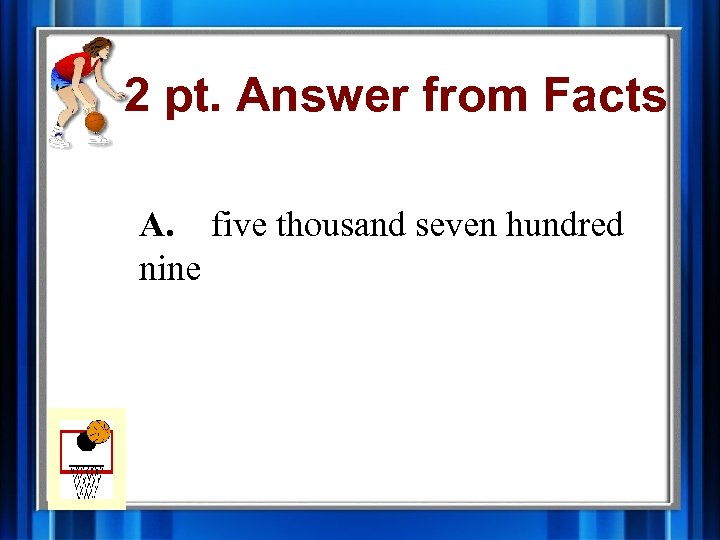2 pt. Answer from Facts A. five thousand seven hundred nine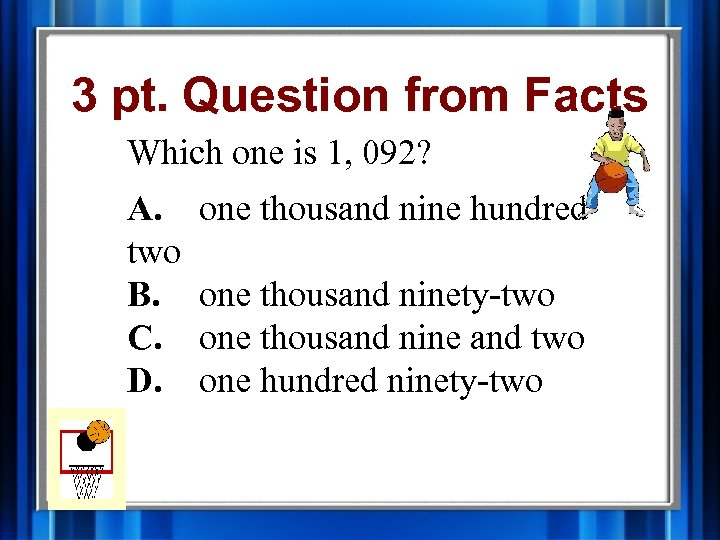3 pt. Question from Facts Which one is 1, 092? A. two B. C. D. one thousand nine hundred one thousand ninety-two one thousand nine and two one hundred ninety-two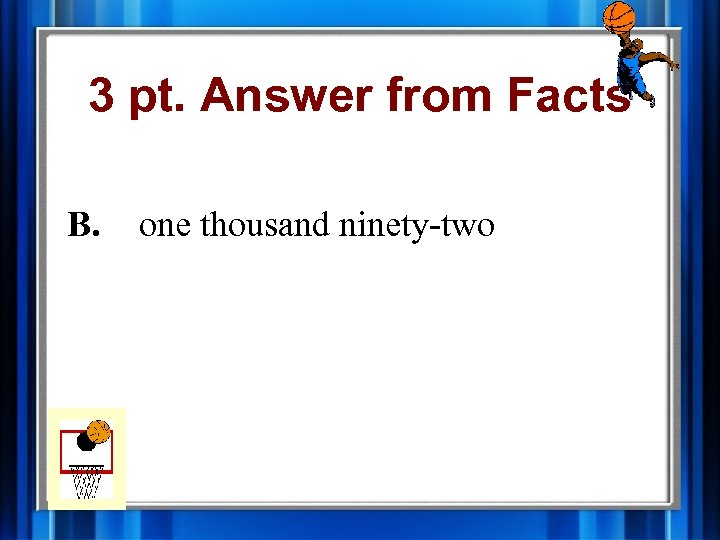3 pt. Answer from Facts B. one thousand ninety-two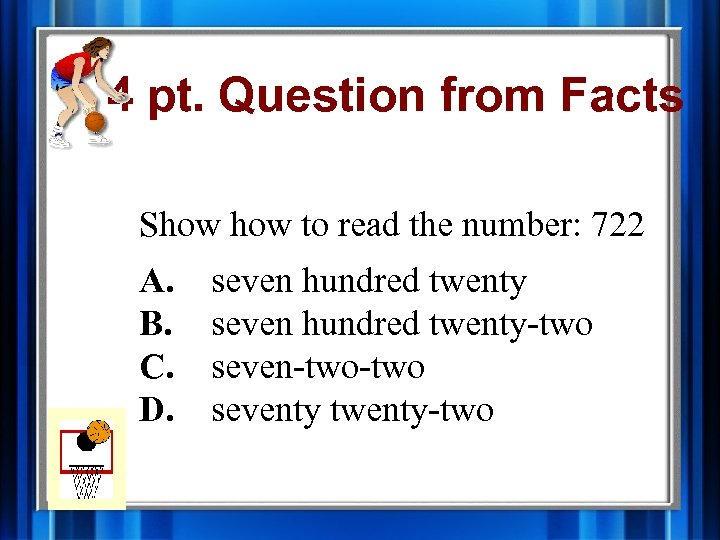4 pt. Question from Facts Show to read the number: 722 A. B. C. D. seven hundred twenty-two seven-two seventy twenty-two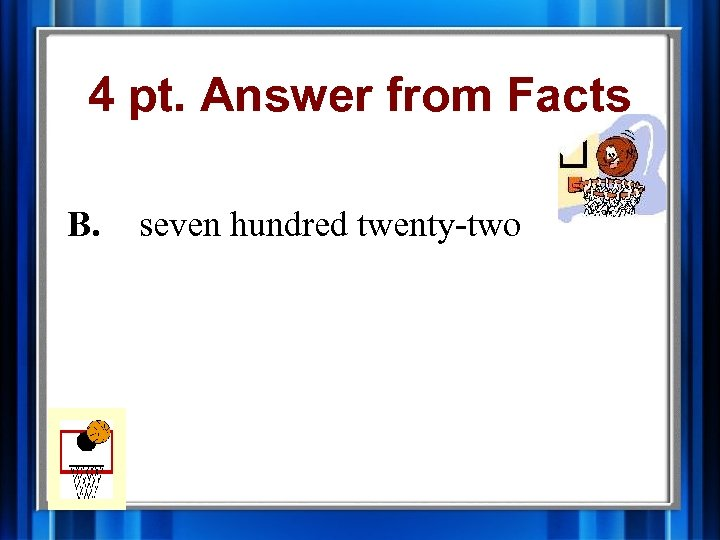4 pt. Answer from Facts B. seven hundred twenty-two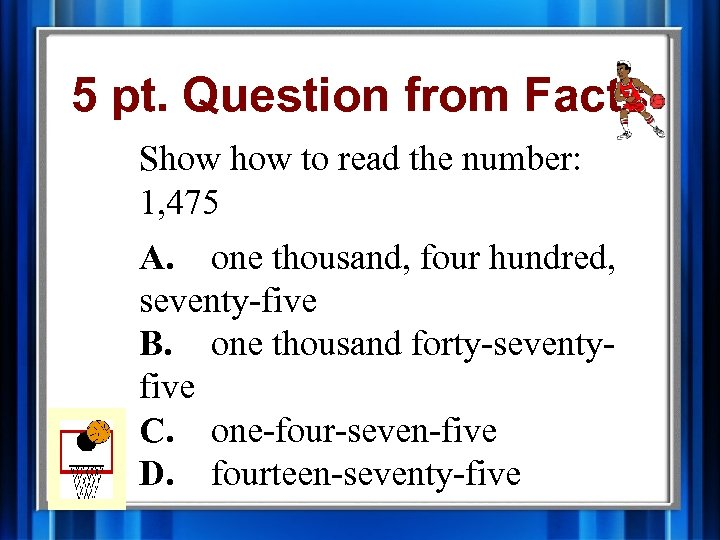5 pt. Question from Facts Show to read the number: 1, 475 A. one thousand, four hundred, seventy-five B. one thousand forty-seventyfive C. one-four-seven-five D. fourteen-seventy-five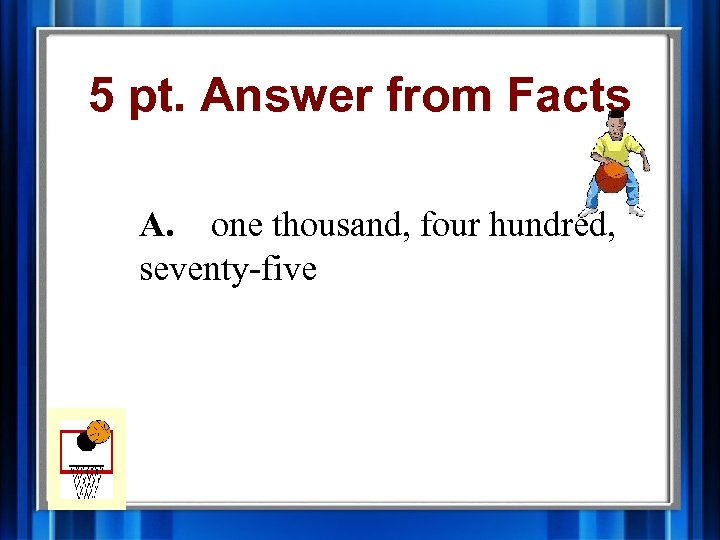5 pt. Answer from Facts A. one thousand, four hundred, seventy-five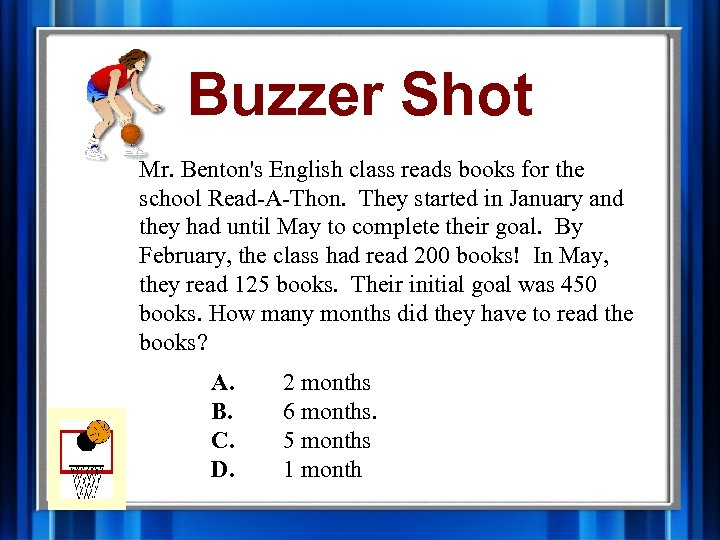Buzzer Shot Mr. Benton's English class reads books for the school Read-A-Thon. They started in January and they had until May to complete their goal. By February, the class had read 200 books! In May, they read 125 books. Their initial goal was 450 books. How many months did they have to read the books? A. B. C. D. 2 months 6 months. 5 months 1 month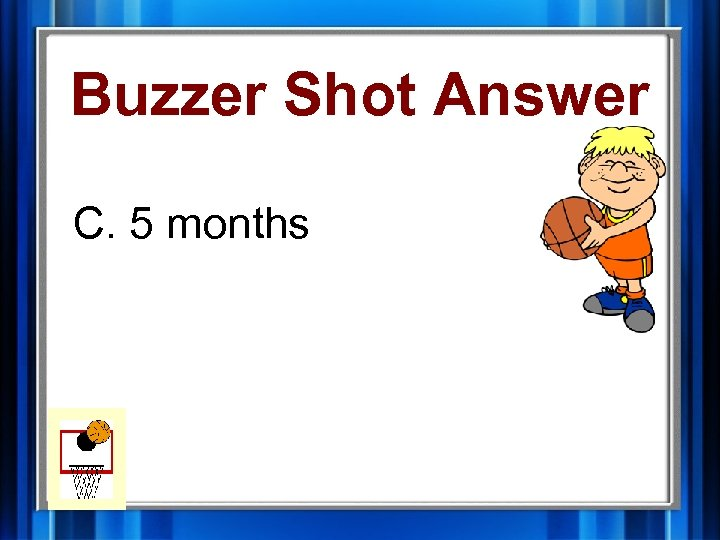Buzzer Shot Answer C. 5 months